# Friction Laws Of Friction Laws Of Motion

Laws Of Friction

Friction is directly proportionate to Normal Force. The law of static friction may thus be written as fs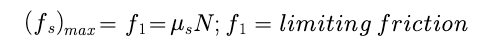Coeficient of limiting Friction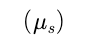depends on nature of two surface in contact and it is indepenedent on area of contact.

Coeficient of kinetic  Friction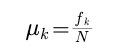It is independent on velocity of body.

Coeficient of rolling  Friction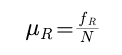Rolling Friction depends on area of the surface in contact.

Note: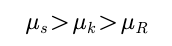Angle of friction : Angle made by resultant of f and N with the normal reaction force is called angle of friction.

Friction is parallel component of contact force to the surfaces.

Normal force is perpendicular component of contact force to the surfaces.

Motion on a horizontal surface.

Consider a block of mass m place on a horizontal surface with Normal Recation N.

Case 1: If applied force F = 0 then Force of Friction is Zero.